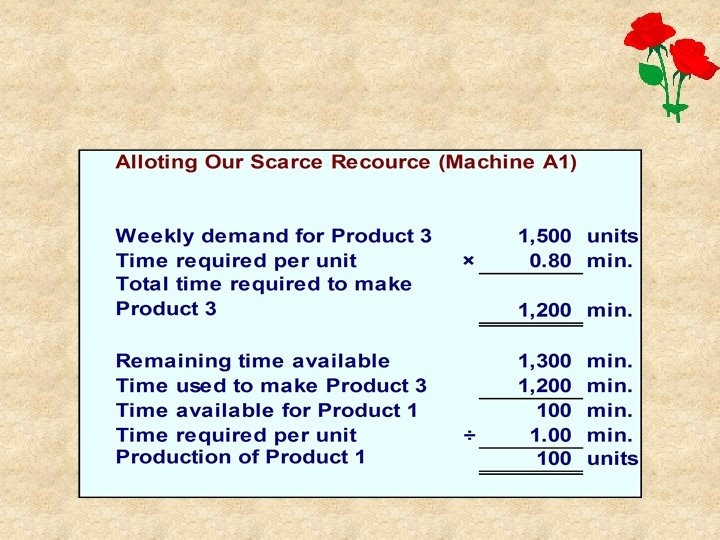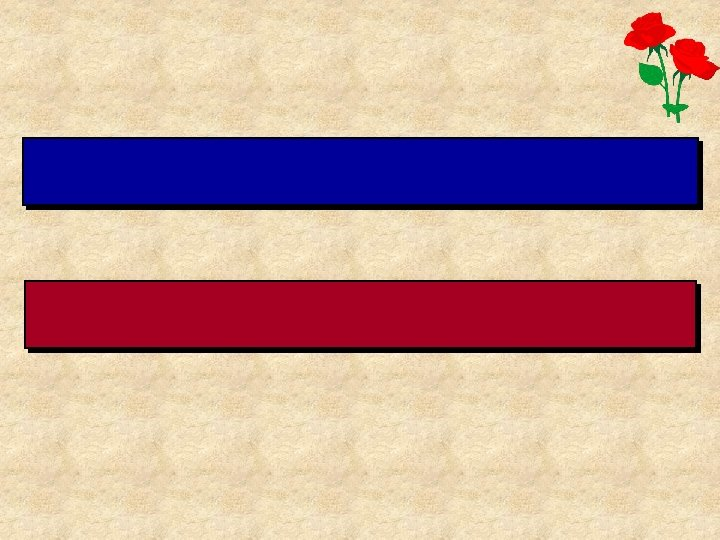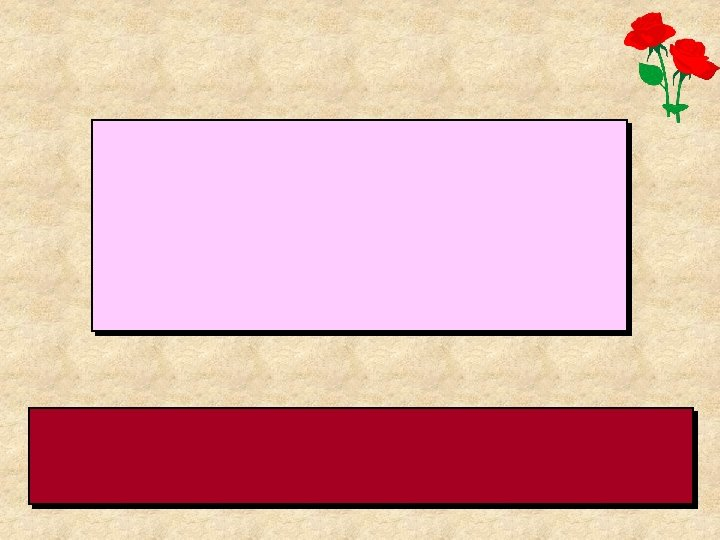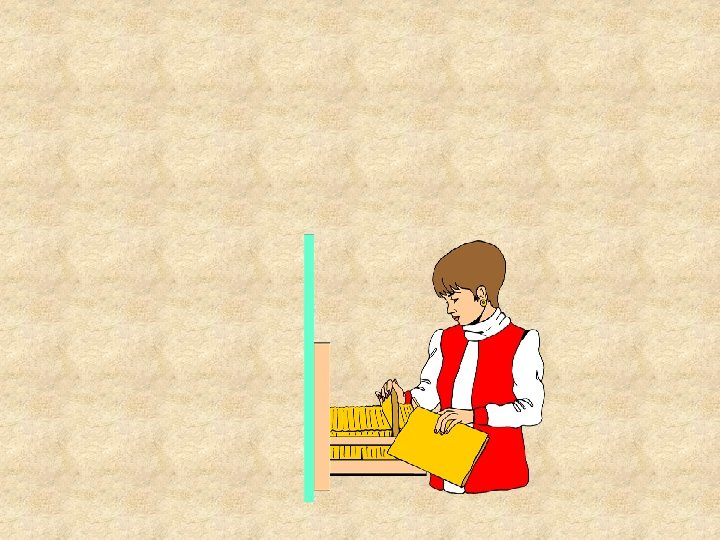Скачать презентацию P V is the unit contribution margin

420e373f87e1361f4ef1361243321e15.ppt

• Количество слайдов: 47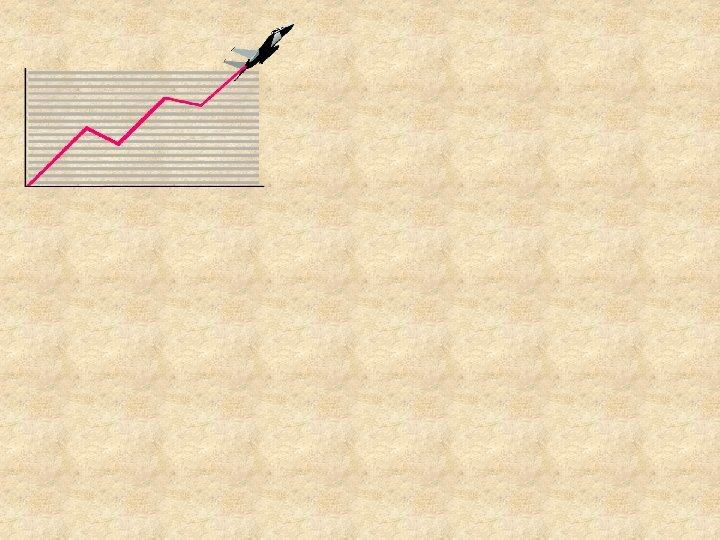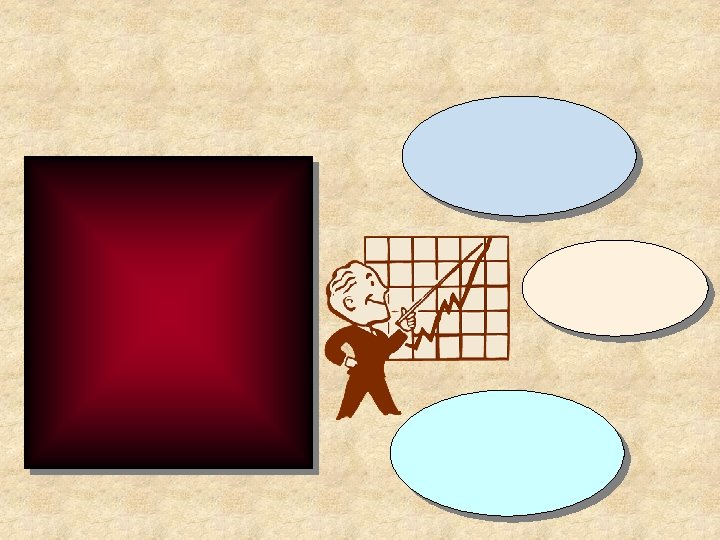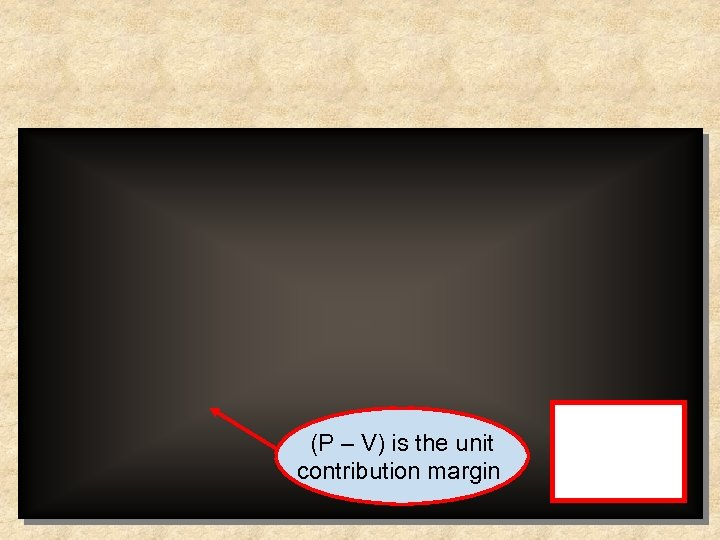(P – V) is the unit contribution margin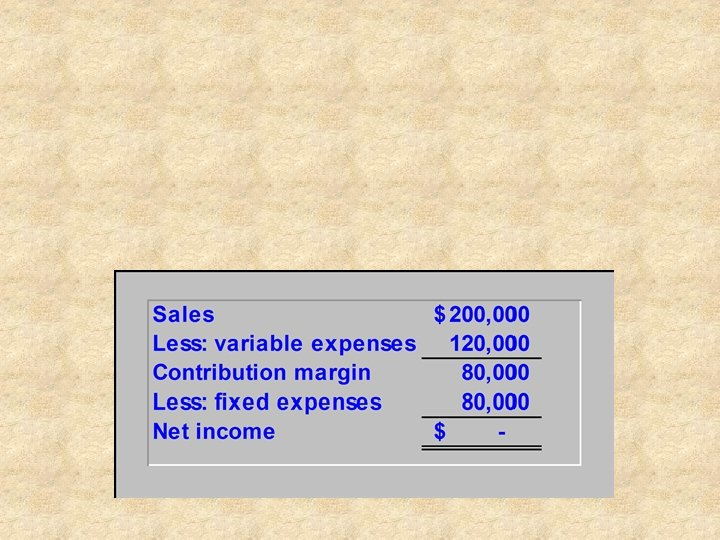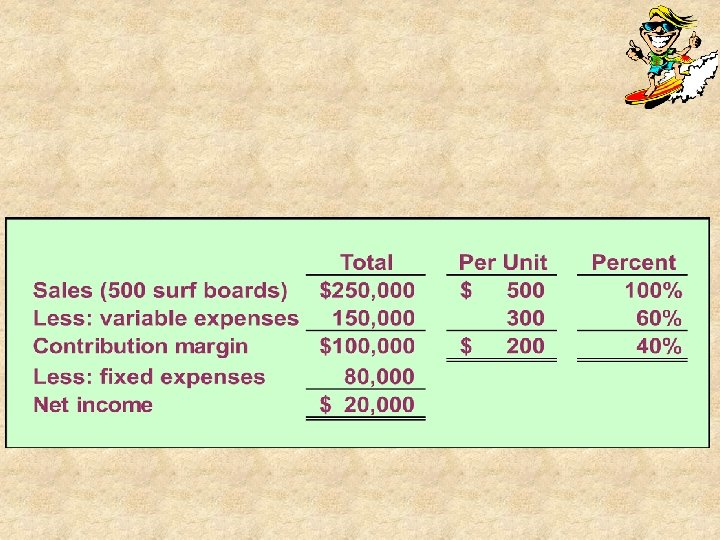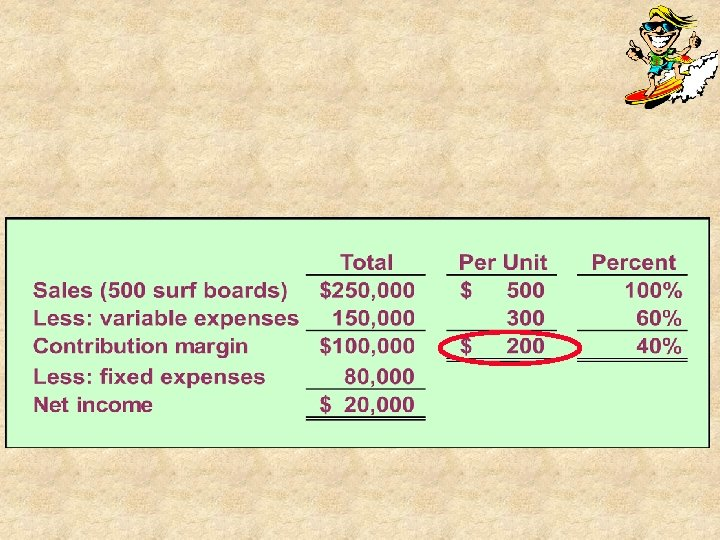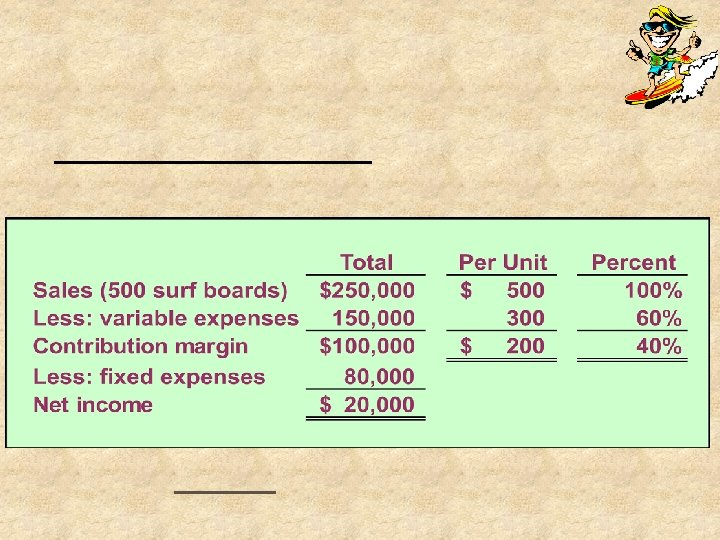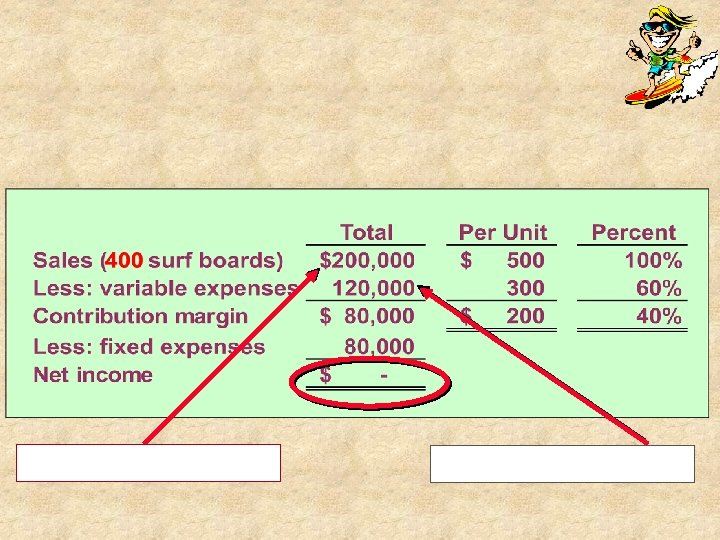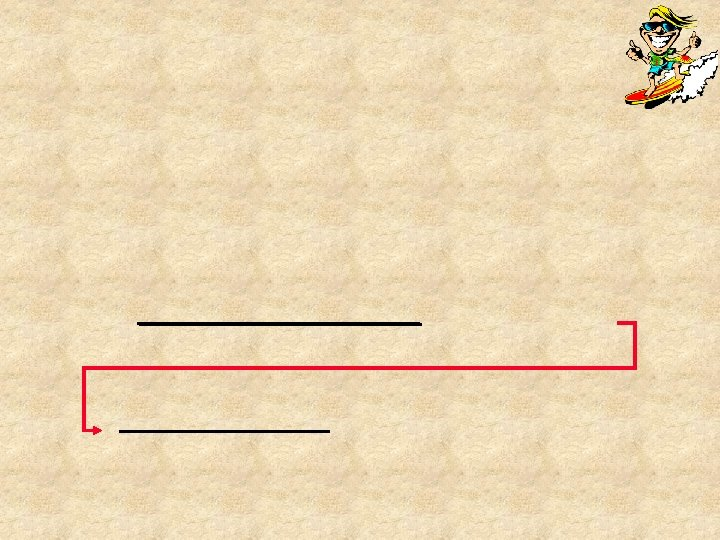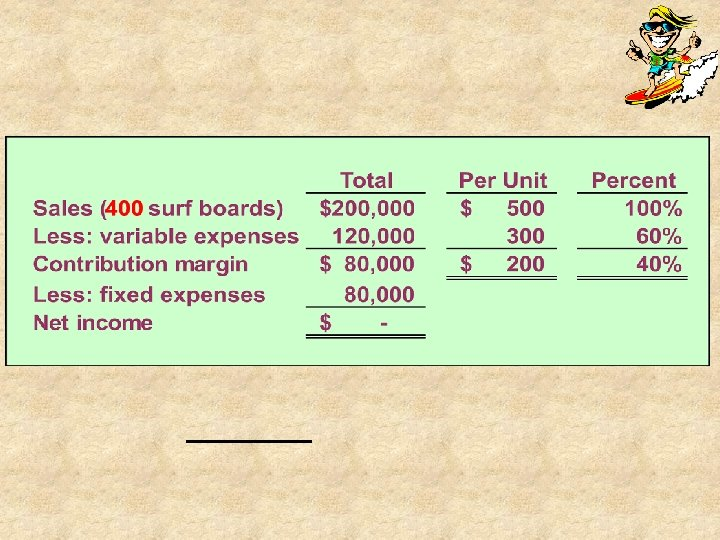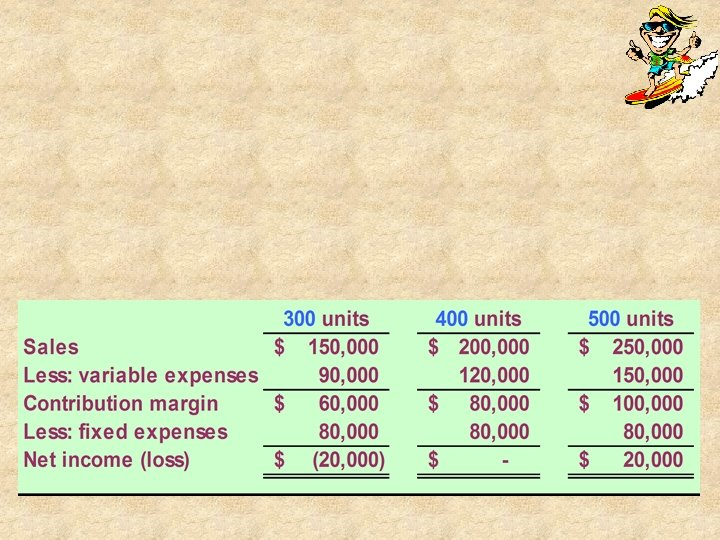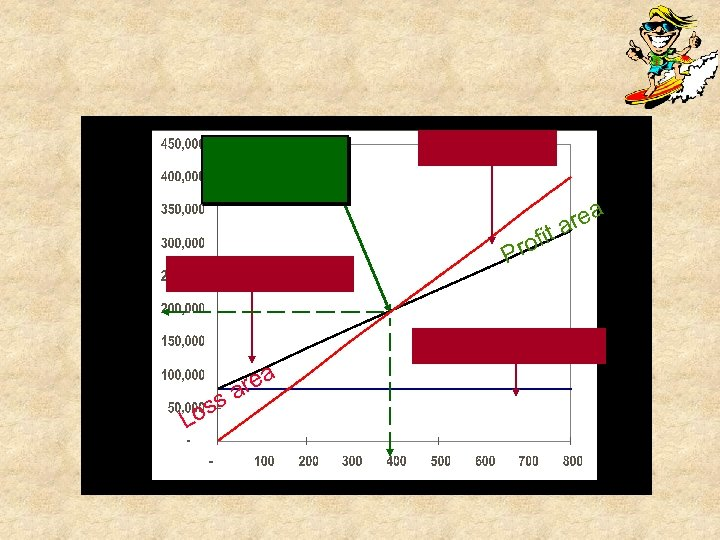ofit Pr s os L rea a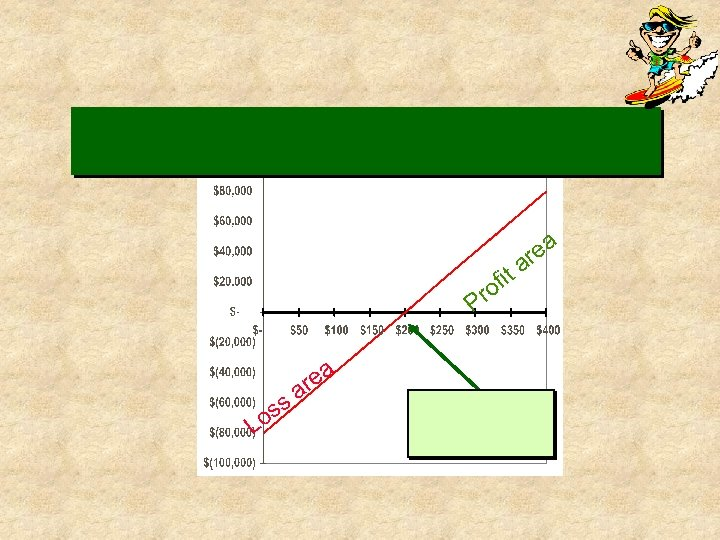ea r ro P ss Lo a re a it a f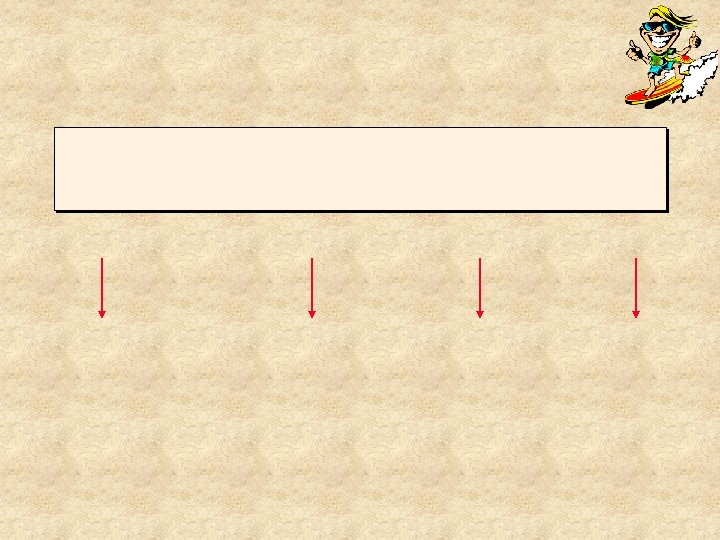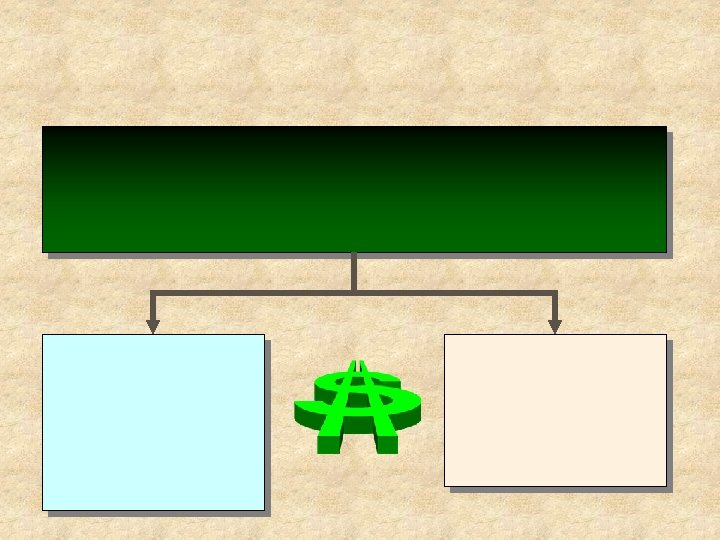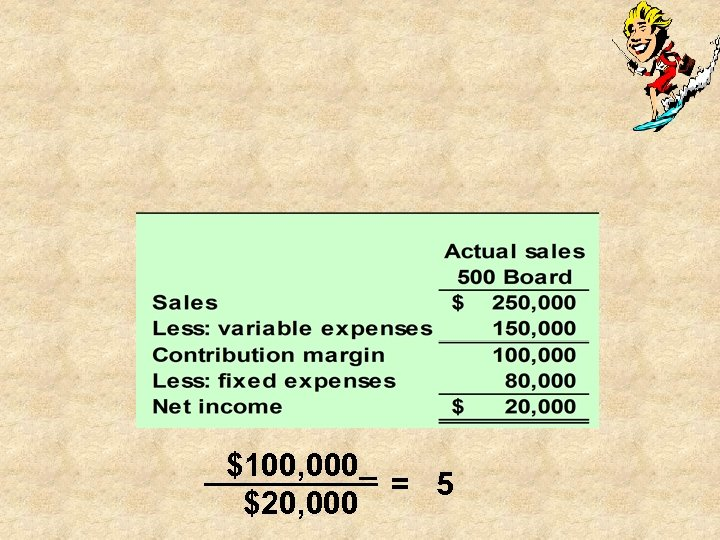\$100, 000 \$20, 000 = 5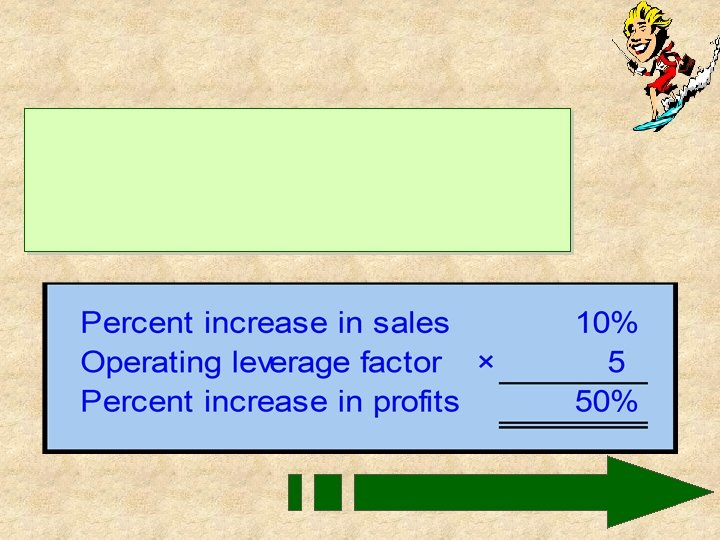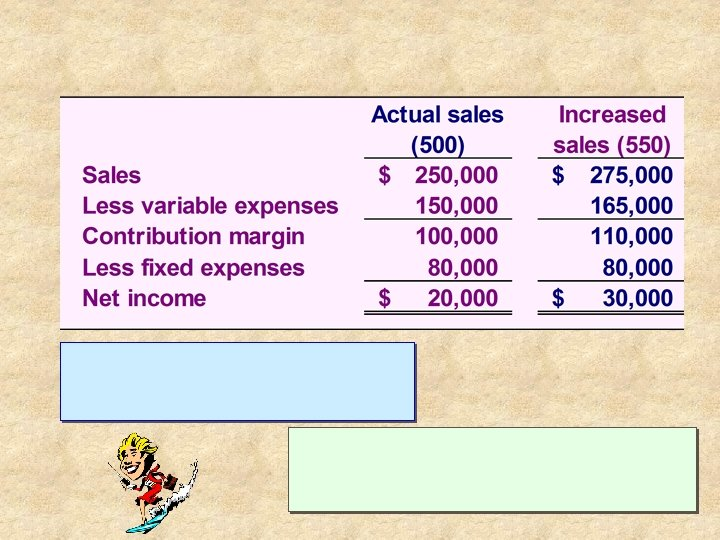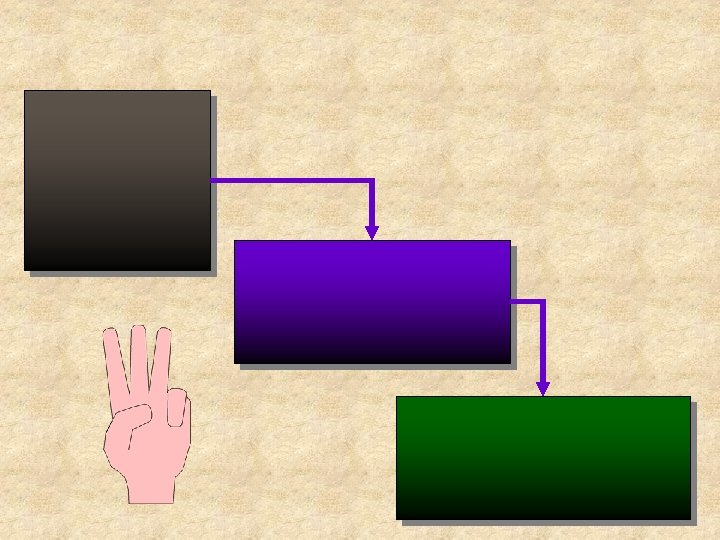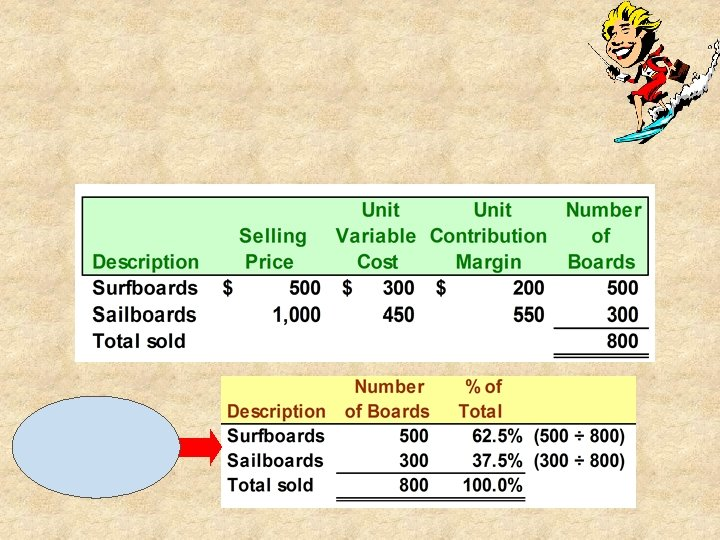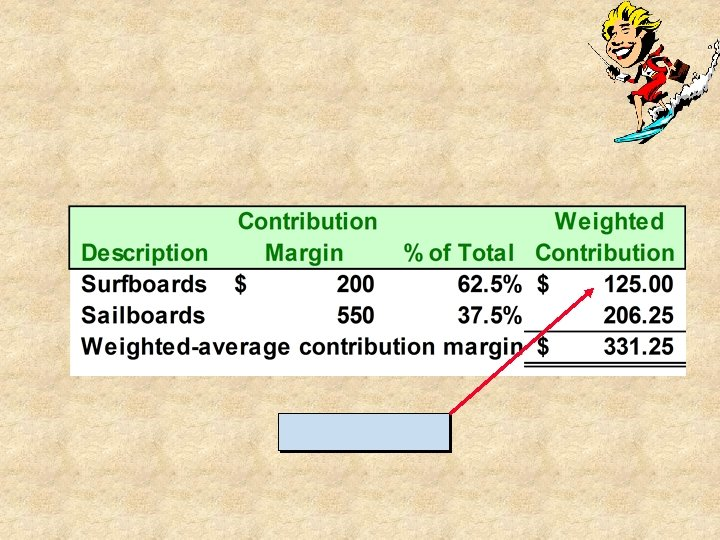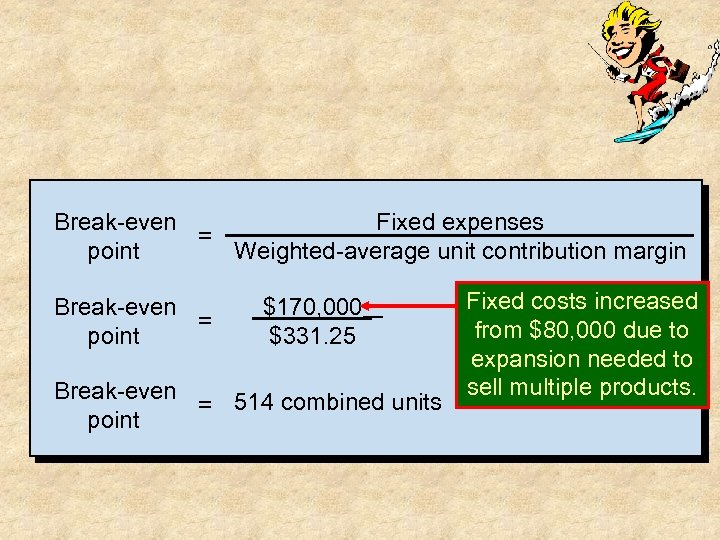Break-even Fixed expenses = point Weighted-average unit contribution margin Break-even = point \$170, 000 \$331. 25 Break-even = 514 combined units point Fixed costs increased from \$80, 000 due to expansion needed to sell multiple products.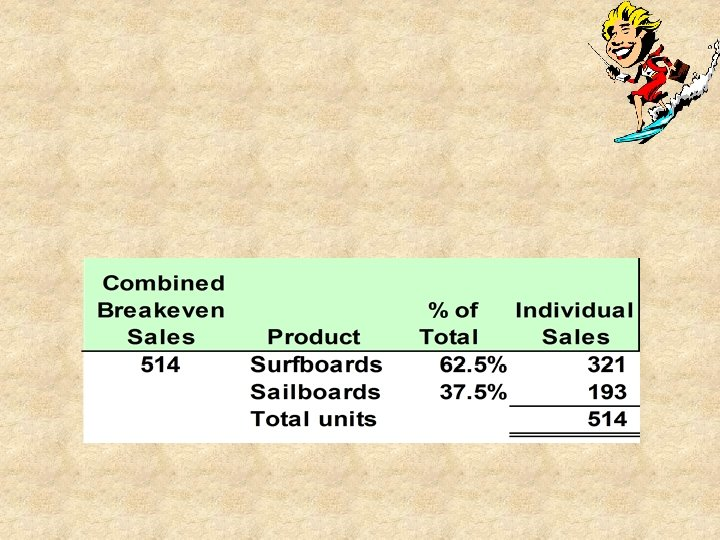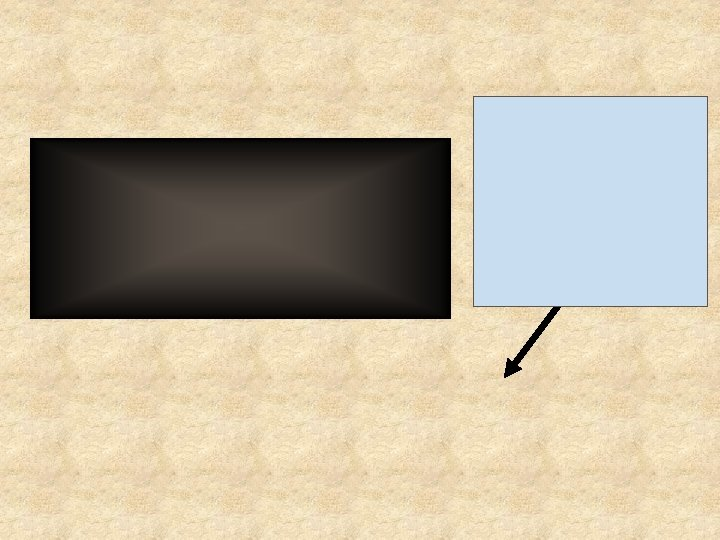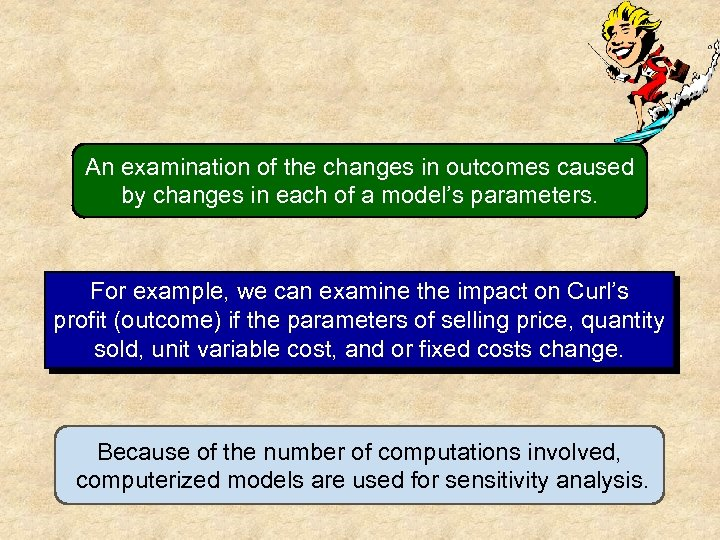An examination of the changes in outcomes caused by changes in each of a model’s parameters. For example, we can examine the impact on Curl’s profit (outcome) if the parameters of selling price, quantity sold, unit variable cost, and or fixed costs change. Because of the number of computations involved, computerized models are used for sensitivity analysis.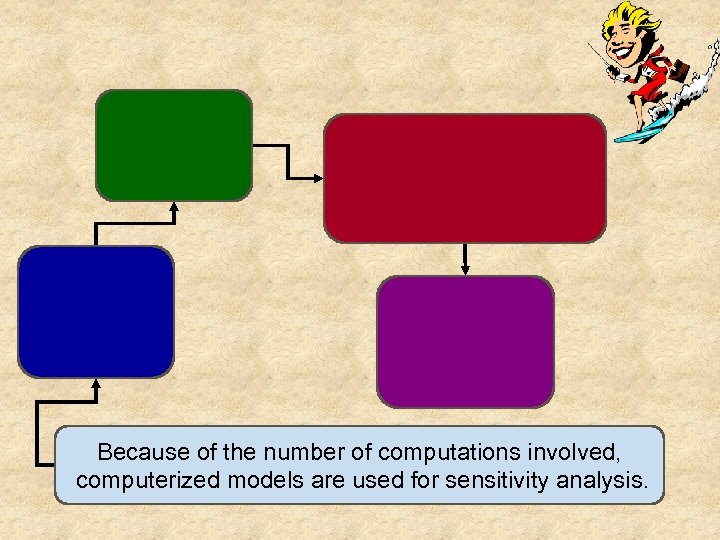Because of the number of computations involved, computerized models are used for sensitivity analysis.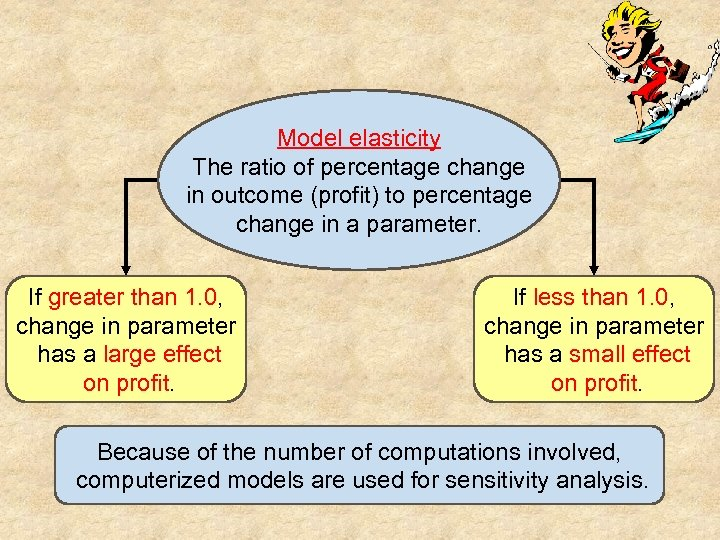Model elasticity The ratio of percentage change in outcome (profit) to percentage change in a parameter. If greater than 1. 0, change in parameter has a large effect on profit. If less than 1. 0, change in parameter has a small effect on profit. Because of the number of computations involved, computerized models are used for sensitivity analysis.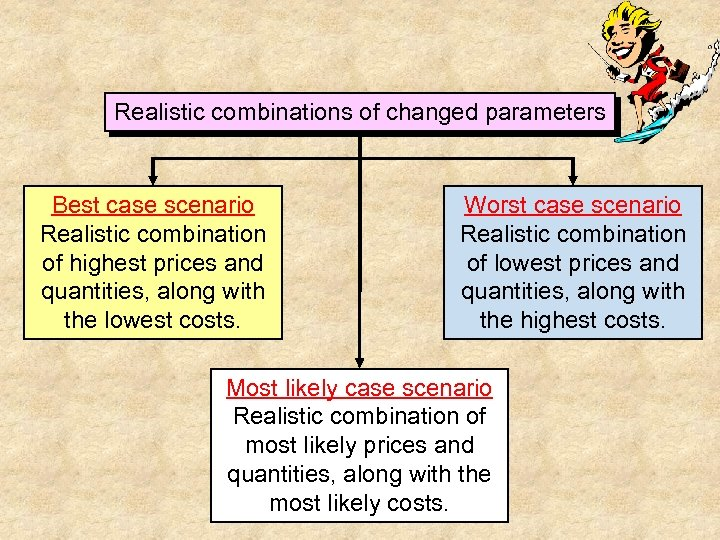Realistic combinations of changed parameters Best case scenario Realistic combination of highest prices and quantities, along with the lowest costs. Worst case scenario Realistic combination of lowest prices and quantities, along with the highest costs. Most likely case scenario Realistic combination of most likely prices and quantities, along with the most likely costs.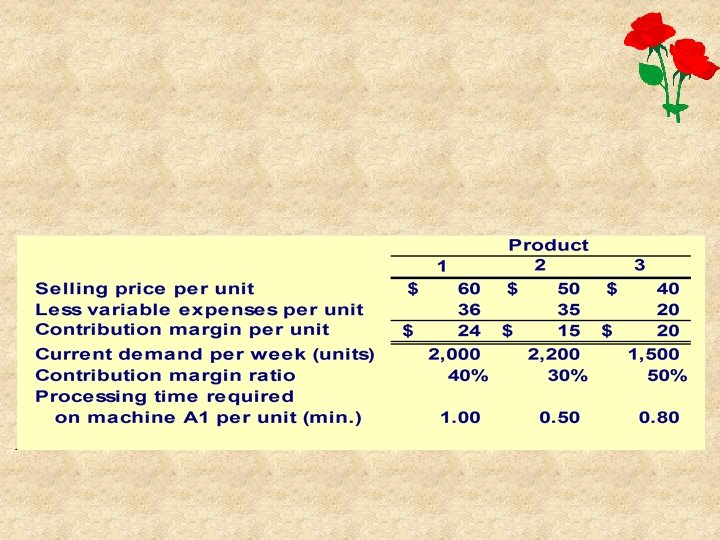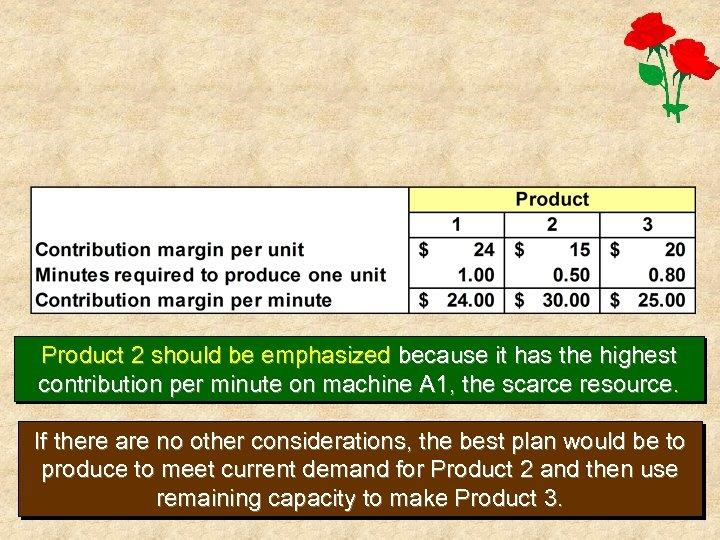Product 2 should be emphasized because it has the highest contribution per minute on machine A 1, the scarce resource. If there are no other considerations, the best plan would be to produce to meet current demand for Product 2 and then use remaining capacity to make Product 3.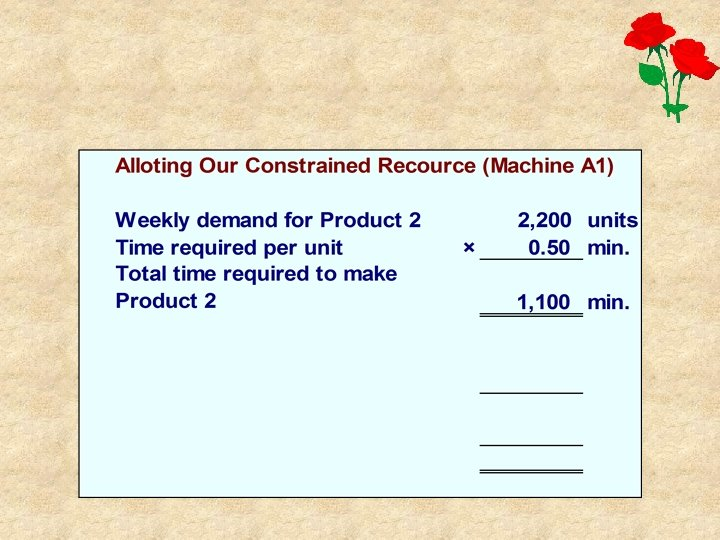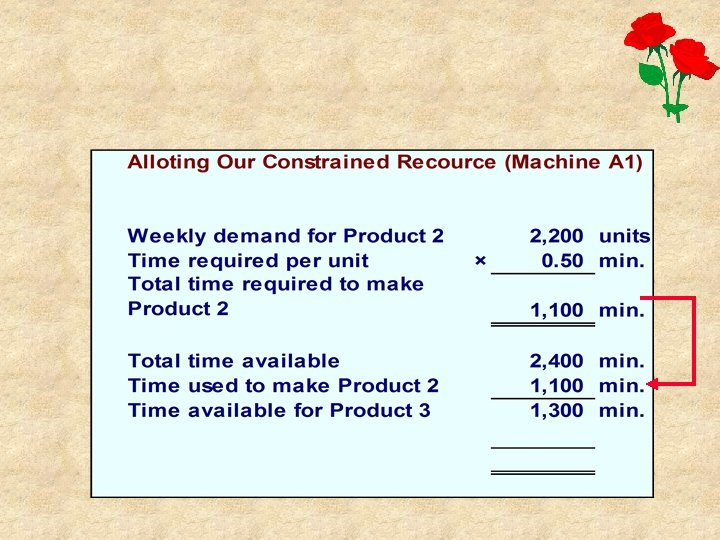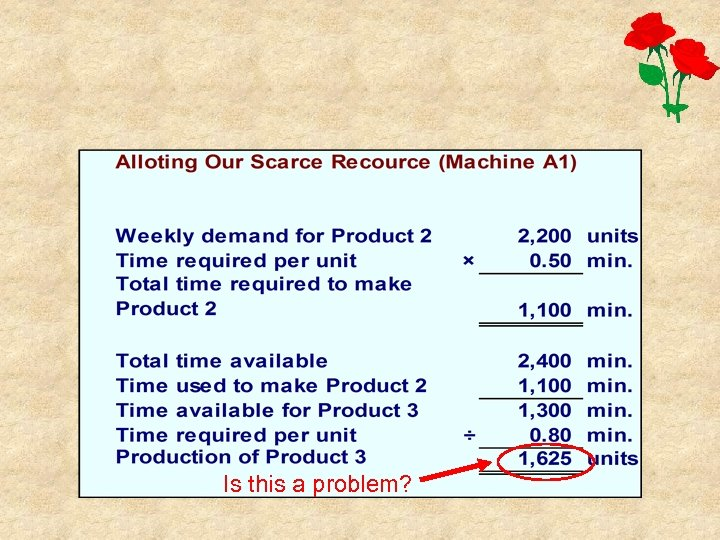Is this a problem?